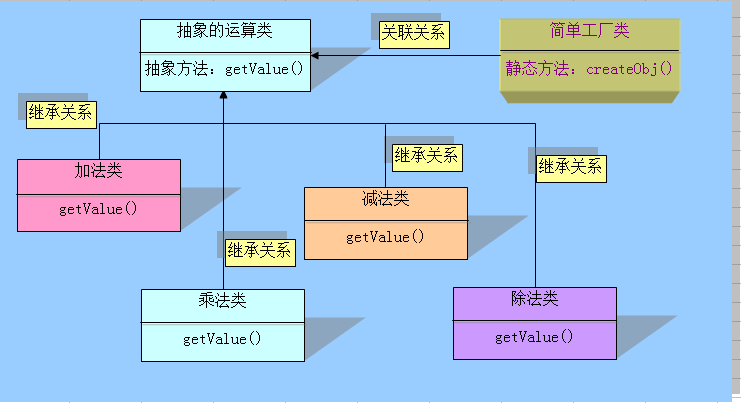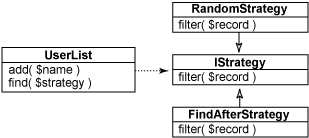# php 设计模式

1.单例模式

1. 一是某个类只能有一个实例；
2. 二是它必须自行创建这个实例；
3. 三是它必须自行向整个系统提供这个实例。

1. 1. php的应用主要在于数据库应用, 一个应用中会存在大量的数据库操作, 在使用面向对象的方式开发时, 如果使用单例模式, 则可以避免大量的new 操作消耗的资源,还可以减少数据库连接这样就不容易出现 too many connections情况。
2. 2. 如果系统中需要有一个类来全局控制某些配置信息, 那么使用单例模式可以很方便的实现. 这个可以参看zend Framework的FrontController部分。
3. 3. 在一次页面请求中, 便于进行调试, 因为所有的代码(例如数据库操作类db)都集中在一个类中, 我们可以在类中设置钩子, 输出日志，从而避免到处var_dump, echo。

例子：

/**
* 设计模式之单例模式
* $_instance必须声明为静态的私有变量 * 构造函数必须声明为私有,防止外部程序new类从而失去单例模式的意义 * getInstance()方法必须设置为公有的,必须调用此方法以返回实例的一个引用 * ::操作符只能访问静态变量和静态函数 * new对象都会消耗内存 * 使用场景:最常用的地方是数据库连接。 * 使用单例模式生成一个对象后，该对象可以被其它众多对象所使用。 */ class man { //保存例实例在此属性中 private static$_instance;

//构造函数声明为private,防止直接创建对象
private function __construct()
{
echo '我被实例化了！';
}

//单例方法
public static function get_instance()
{
var_dump(isset(self::$_instance)); if(!isset(self::$_instance))
{
self::$_instance=new self(); } return self::$_instance;
}

//阻止用户复制对象实例
private function __clone()
{
trigger_error('Clone is not allow' ,E_USER_ERROR);
}

function test()
{
echo("test");

}
}

// 这个写法会出错，因为构造方法被声明为private
//$test = new man; // 下面将得到Example类的单例对象$test = man::get_instance();
$test = man::get_instance();$test->test();

// 复制对象将导致一个E_USER_ERROR.
//$test_clone = clone$test;

2.简单工厂模式
• ①抽象基类：类中定义抽象一些方法，用以在子类中实现
• ②继承自抽象基类的子类：实现基类中的抽象方法
• ③工厂类：用以实例化所有相对应的子类/**
*
* 定义个抽象的类，让子类去继承实现它
*

*/
abstract class Operation{
//抽象方法不能包含函数体
abstract public function getValue($num1,$num2);//强烈要求子类必须实现该功能函数
}

/**
* 加法类

*/
public function getValue($num1,$num2){
return $num1+$num2;
}
}
/**
* 减法类

*/
class OperationSub extends Operation {
public function getValue($num1,$num2){
return $num1-$num2;
}
}
/**
* 乘法类

*/
class OperationMul extends Operation {
public function getValue($num1,$num2){
return $num1*$num2;
}
}
/**
* 除法类

*/
class OperationDiv extends Operation {
public function getValue($num1,$num2){
try {
if ($num2==0){ throw new Exception("除数不能为0"); }else { return$num1/$num2; } }catch (Exception$e){
echo "错误信息：".$e->getMessage(); } } } 通过采用面向对象的继承特性，我们可以很容易就能对原有程序进行扩展，比如:‘乘方’，‘开方’，‘对数’，‘三角函数’，‘统计’等，以还可以避免加载没有必要的代码。 如果我们现在需要增加一个求余的类，会非常的简单 我们只需要另外写一个类（该类继承虚拟基类）,在类中完成相应的功能（比如：求乘方的运算）,而且大大的降低了耦合度，方便日后的维护及扩展 /** * 求余类（remainder） * */ class OperationRem extends Operation { public function getValue($num1,$num2){ return$num1%$num12; } } 现在还有一个问题未解决,就是如何让程序根据用户输入的操作符实例化相应的对象呢？ 解决办法：使用一个单独的类来实现实例化的过程，这个类就是工厂 /** * 工程类，主要用来创建对象 * 功能：根据输入的运算符号，工厂就能实例化出合适的对象 * */ class Factory{ public static function createObj($operate){
switch ($operate){ case '+': return new OperationAdd(); break; case '-': return new OperationSub(); break; case '*': return new OperationSub(); break; case '/': return new OperationDiv(); break; } } }$test=Factory::createObj('/');
$result=$test->getValue(23,0);
echo $result; 其他关于关于此模式的笔记： 工厂模式： 以交通工具为例子：要求请既可以定制交通工具，又可以定制交通工具生产的过程 1>定制交通工具 1.定义一个接口，里面包含交工工具的方法（启动 运行 停止） 2.让飞机，汽车等类去实现他们 2> 定制工厂（通上类似） 1.定义一个接口，里面包含交工工具的制造方法（启动 运行 停止） 2.分别写制造飞机，汽车的工厂类去继承实现这个接口 3.观察者模式 观察者模式属于行为模式，是定义对象间的一种一对多的依赖关系，以便当一个对象的状态发生改变时，所有依 赖于它的对象都得到通知并自动刷新。它完美的将观察者对象和被观察者对象分离。可以在独立的对象（主体）中维护一个对主体感兴趣的依赖项（观察器）列表。 让所有观察器各自实现公共的 Observer 接口，以取消主体和依赖性对象之间的直接依赖关系。（反正我看不明白） 用到了 spl （standard php library） class MyObserver1 implements SplObserver { public function update(SplSubject$subject) {
echo __CLASS__ . ' - ' . $subject->getName(); } } class MyObserver2 implements SplObserver { public function update(SplSubject$subject) {
echo __CLASS__ . ' - ' . $subject->getName(); } } class MySubject implements SplSubject { private$_observers;
private $_name; public function __construct($name) {
$this->_observers = new SplObjectStorage();$this->_name = $name; } public function attach(SplObserver$observer) {
$this->_observers->attach($observer);
}

public function detach(SplObserver $observer) {$this->_observers->detach($observer); } public function notify() { foreach ($this->_observers as $observer) {$observer->update($this); } } public function getName() { return$this->_name;
}
}

$observer1 = new MyObserver1();$observer2 = new MyObserver2();

$subject = new MySubject("test");$subject->attach($observer1);$subject->attach($observer2);$subject->notify();

4.策略模式

在此模式中，算法是从复杂类提取的，因而可以方便地替换。例如，如果要更改搜索引擎中排列页的方法，则策略模式是一个不错的选择。思考一下搜索引擎的几个部分 —— 一部分遍历页面，一部分对每页排列，另一部分基于排列的结果排序。在复杂的示例中，这些部分都在同一个类中。通过使用策略模式，您可将排列部分放入另一个类中，以便更改页排列的方式，而不影响搜索引擎的其余代码。作为一个较简单的示例，下面 显示了一个用户列表类，它提供了一个根据一组即插即用的策略查找一组用户的方法

//定义接口
interface IStrategy {
function filter($record); } //实现接口方式1 class FindAfterStrategy implements IStrategy { private$_name;
public function __construct($name) {$this->_name = $name; } public function filter($record) {
return strcmp ( $this->_name,$record ) <= 0;
}
}

//实现接口方式1
class RandomStrategy implements IStrategy {
public function filter($record) { return rand ( 0, 1 ) >= 0.5; } } //主类 class UserList { private$_list = array ();
public function __construct($names) { if ($names != null) {
foreach ( $names as$name ) {
$this->_list [] =$name;
}
}
}

public function add($name) {$this->_list [] = $name; } public function find($filter) {
$recs = array (); foreach ($this->_list as $user ) { if ($filter->filter ( $user ))$recs [] = $user; } return$recs;
}
}

$ul = new UserList ( array ( "Andy", "Jack", "Lori", "Megan" ) );$f1 = $ul->find ( new FindAfterStrategy ( "J" ) ); print_r ($f1 );

$f2 =$ul->find ( new RandomStrategy () );
print_r ( \$f2 );

posted on 2012-09-09 13:56  思齐_  阅读(54714)  评论(12编辑  收藏  举报